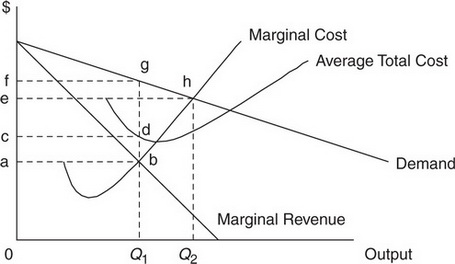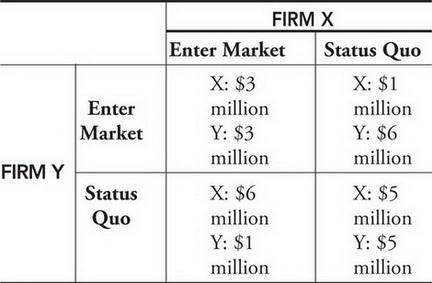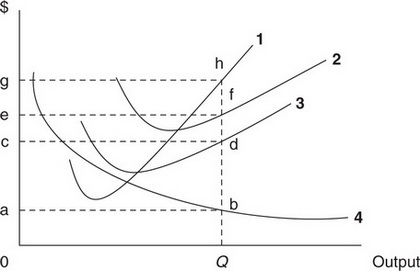# AP Microeconomics Practice Test 16

### Test Information12 questions14 minutes

1. Which of the following might explain how a price decrease might cause a decrease in quantity demanded and an upward-sloping demand curve?

2. For the perfectly competitive firm, the profit-maximizing decision to shut down is made when the price

3. Declining populations of tuna in the Atlantic Ocean have likely had which of the following impacts on the wages of tuna fishermen, the employment of tuna fishermen, and real estate prices in New England fishing towns?

FISHERMAN WAGES     EMPLOYMENT OF FISHERMEN     REAL ESTATE PRICES

4. Which of the following is true of monopoly markets?

5.If this firm were a profit-maximizing monopolist, the price and output would be which of the following?

6.Deadweight loss is equal to which of the following areas?

7. Two competing firms are deciding whether to enter a new market or maintain the status quo. Use the following profit matrix to respond to question below.If these firms do not collude, the outcome will be

8. When the marginal product of labor is equal to the average product of labor,

9.The area 0abQ is equal to

10.The curve labeled 1 represents which of the following?

11.If this firm was operating in a perfectly competitive market, and the price was equal to 0g, economic profit would be equal to which of the following areas?

12. Which is true of monopolistic competition?